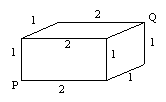## Project 15: Nets of Bricks

Consider the "brick" which is a rectangular solid which is 1x1xm where m is an integer. When m =1 we have the cube and the 1x1x2 brick is illustrated in the figure below. We will think of the brick as a surface rather than as a solid. When we speak of the distance between two points on the brick we mean the distance along (over) the surface of the brick. Points P and Q on an 1x1xm brick are the points that correspond to the points P and Q labeled in the figure below:Problems

1. Determine the possible nets of bricks for different values of m = 1, 2, ....

(Test your intuition: Do you think there are more nets for m=1 or m=2? m=2 or m=3?)

2. Determine the shortest distance between the points P and Q as a function of m, the size of the brick.

3. If the point P is fixed, what is the farthest point on the brick from P? (If there are many points which can be farthest from P give a description of the set of possible points.)

4. What is the largest distance between any two points on the the brick? (Can you give a formula for this distance as a function of m?

Extensions

1. Nothing in the description above requires that m be an integer. Do any differences in behavior occur for bricks where m is not an integer?

Joseph Malkevitch
Department of Mathematics and Computing
York College (CUNY)
Jamaica, New York 11451-0001
email: malkevitch@york.cuny.edu
(Comments and results related to the project above are welcome.)

Acknowledgements
Some of this work was prepared with partial support from the National Science Foundation (Grant Number: DUE 9555401) to the Long Island Consortium for Interconnected Learning (administered by SUNY at Stony Brook, Alan Tucker, Project Director).